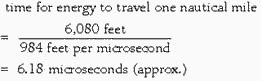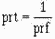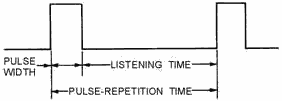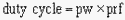Please Support RF Cafe by purchasing my  ridiculously low−priced products, all of which I created.

RF Workbench  (shareware)# Navy Electricity and Electronics Training Series (NEETS)Module 18 - Radar PrinciplesChapter 1:  Pages 1-1 through 1-10

Chapter 1

Learning Objectives

Learning objectives are stated at the beginning of each chapter. These learning objectives serve as a preview of the information you are expected to learn in the chapter. The comprehensive check questions are based on the objectives. By successfully completing the OCC/ECC, you indicate that you have met the objectives and have learned the information. The learning objectives are listed below.

1. Define range, bearing, and altitude as they relate to a radar system.

2. Discuss how pulse width, peak power, and beam width affect radar performance.

3. Describe the factors that contribute to or detract from radar accuracy.

4. Using a block diagram, describe the basic function, principles of operation, and interrelationships of the basic units of a radar system.

5. Explain the various ways in which radar systems are classified, including the standard

Army/Navy classification system.

6. Explain the basic operation of cw, pulse, and Doppler radar systems.

The term Radar is common in today's everyday language. You probably use it yourself when referring to a method of recording the speed of a moving object. The term Radar is an acronym made up of the words radio detection and ranging. The term is used to refer to electronic equipment that detect the presence, direction, height, and distance of objects by using reflected electromagnetic energy. Electromagnetic energy of the frequency used for radar is unaffected by darkness and also penetrates weather to some degree, depending on frequency. It permits radar systems to determine the positions of ships, planes, and land masses that are invisible to the naked eye because of distance, darkness, or weather.

The develoPMent of radar into the highly complex systems in use today represents the accumulated develoPMents of many people and nations. The general principles of radar have been known for a long time, but many electronics discoveries were necessary before a useful radar system could be developed. World War II provided a strong incentive to develop practical radar, and early versions were in use soon after the war began. Radar technology has improved in the years since the war. We now have radar systems that are smaller, more efficient, and better than those early versions.

Modern radar systems are used for early detection of surface or air objects and provide extremely accurate information on distance, direction, height, and speed of the objects. Radar is also used to guide missiles to targets and direct the firing of gun systems. Other types of radar provide long-distance surveillance and navigation information.

The electronics principle on which radar operates is very similar to the principle of sound-wave reflection. If you shout in the direction of a sound-reflecting object (like a rocky canyon or cave), you will hear an echo. If you know the speed of sound in air, you can then estimate the distance and general direction of the object. The time required for a return echo can be roughly converted to distance if the speed of sound is known. Radar uses electromagnetic energy pulses in much the same way, as shown in figure 1-1. The radio-frequency (RF) energy is transmitted to and reflects from the reflecting object. a small portion of the energy is reflected and returns to the radar set. This returned energy is called an ECHO, just as it is in sound terminology. Radar sets use the echo to determine the direction and distance of the reflecting object.Note: The terms TARGET, RETurn, ECHO, CONTACT, OBJECT, and REFLECTING OBJECT are used interchangeably throughout this module to indicate a surface or airborne object that has been detected by a radar system.

Radar systems also have some characteristics in common with telescopes. Both provide only a limited field of view and require reference coordinate systems to define the positions of detected objects. If you describe the location of an object as you see it through a telescope, you will most likely refer to prominent features of the landscape. Radar requires a more precise reference system. Radar surface angular measurements are normally made in a clockwise direction from TRUE NORTH, as shown in figure 1-2, or from the heading line of a ship or aircraft. The surface of the earth is represented by an imaginary flat plane, tangent (or parallel) to the earth's surface at that location. This plane is referred to as the HORIZONTAL Plane. All angles in the up direction are measured in a second imaginary plane that is perpendicular to the horizontal plane.Figure 1-2. - Radar reference coordinates.

This second plane is called the VERTICAL Plane. The radar location is the center of this coordinate system. The line from the radar set directly to the object is referred to as the LINE of SIGHT (los). The length of this line is called RANGE. The angle between the horizontal plane and the los is the ELEVATION ANGLE. The angle measured clockwise from true north in the horizontal plane is called the TRUE BEARING or AZIMUTH angle. These three coordinates of range, bearing, and elevation describe the location of an object with respect to the antenna.

Q1. Radar surface-angular measurements are referenced to true north and measured in what plane?

Q2. The distance from a radar set to a target measured along the line of sight is identified by what

term?

RANGE

Radar measurement of range, or distance, is made possible because of the properties of radiated electromagnetic energy. This energy normally travels through space in a straight line, at a constant speed, and will vary only slightly because of atmospheric and weather conditions. The effects atmosphere and weather have on this energy will be discussed later in this chapter; however, for this discussion on determining range, these effects will be temporarily ignored.

Electromagnetic energy travels through air at approximately the speed of light, which is 186,000

STATUTE MILES per second. The Navy uses NAUTICAL MILES to calculate distances; 186,000 statute miles is approximately 162,000 nautical miles. While the distance of the statute mile is approximately 5,280 feet, the distance for a nautical mile is approximately 6,080 feet.

Radar timing is usually expressed in microseconds. To relate radar timing to distances traveled by radar energy, you should know that radiated energy from a radar set travels at approximately 984 feet per microsecond. With the knowledge that a nautical mile is approximately 6,080 feet, we can figure the approximate time required for radar energy to travel one nautical mile using the following calculation:The same answer can be obtained using yards instead of feet. In the following calculation, the 6,080 foot approximation of a nautical mile is converted to 2,027 yards and energy speed is changed from 984 feet to 328 yards per microsecond:A pulse-type radar set transmits a short burst of electromagnetic energy. Target range is determined by measuring elapsed time while the pulse travels to and returns from the target. Because two-way travel

is involved, a total time of 12.36 (6.18 x 2) microseconds per nautical mile will elapse between the start of the pulse from the antenna and its return to the antenna from a target. This 12.36 microsecond time

interval is sometimes referred to as a Radar MILE, Radar NAUTICAL MILE, or NAUTICAL Radar MILE. The range in nautical miles to an object can be found by measuring the elapsed time during a round trip of a radar pulse and dividing this quantity by 12.36. In equation form, this is:For example, if the elapsed time for an echo is 62 microseconds, then the distance is 5 miles, as shown in the following calculation:Note: Unless otherwise stated all distances will be expressed as nautical miles throughout this module.

Minimum Range

Recall from NEETS, Module 11, Microwave Principles, that the DUPLEXER alternately switches the antenna between the transmitter and receiver so that only one antenna need be used. This switching is necessary because the high-power pulses of the transmitter would destroy the receiver if energy were allowed to enter the receiver. As you probably already realize, timing of this switching action is critical to the operation of the radar system. What you may not realize is that the minimum range ability of the radar system is also affected by this timing. The two most important times in this action are PULSE WIDTH and RECOVery TIME.

This timing action must be such that during the transmitted pulse (pulse width), only the transmitter can be connected to the antenna. Immediately after the pulse is transmitted, the antenna must be reconnected to the receiver.

The leading edge of the transmitted pulse causes the duplexer to align the antenna to the transmitter. This action is essentially instantaneous. At the end of the transmitted pulse, the trailing edge of the pulse causes the duplexer to line up the antenna with the receiver; however, this action is not instantaneous. a small amount of time elapses at this point that is referred to as recovery time. Therefore, the total time in which the receiver is unable to receive the reflected pulse is equal to the pulse width plus the recovery time. Note that any reflected pulses from close targets returning before the receiver is connected to the antenna will be undetected. The minimum range, in yards, at which a target can be detected is determined using the following formula (pulse width and recovery time are expressed in microseconds or fractions of microseconds):For example, minimum range for a radar system with a pulse width of 25 microseconds and a recovery time of 0.1 microseconds is figured as follows:Most modern radar systems are designed with such small recovery times that this figure can often be ignored when figuring minimum range.

Maximum Range

The maximum range of a pulse radar system depends upon CARRIER Frequency, PEAK Power of the transmitted pulse, PULSE-REPETITION Frequency (prf) or PULSE REPETITION RATE (prr), and RECEIVER SENSITIVITY with PRF as the primary limiting factor. The peak power of the pulse determines what maximum range the pulse can travel to a target and still return a usable echo. a usable echo is the smallest signal detectable by a receiver system that can be processed and presented on an indicator.

The frequency of the RF energy in the pulse radiated by a radar is referred to as the CARRIER Frequency of the radar system. The carrier frequency is often a limiting factor in the maximum range capability of a radar system because radio frequency energy above 3,000 megahertz is rapidly attenuated by the atmosphere. This decreases the usable range of radio-frequency energy. Therefore, as the carrier frequency is increased, the transmitted power must also be increased to cover the same range. Long-range coverage is more easily achieved at lower frequencies because atmospheric conditions have less effect on low-frequency energy.

Radar systems radiate each pulse at the carrier frequency during transmit time, wait for returning echoes during listening or rest time, and then radiate a second pulse, as shown in figure 1-3. The number of pulses radiated in one second is called the pulse-repetition frequency (prf), or the pulse-repetition rate (prr). The time between the beginning of one pulse and the start of the next pulse is called PULSE- REPETITION TIME (prt) and is equal to the reciprocal of PRF as follows:Figure 1-3. - Radar pulse relationships.

AMBIGUOUS RETurns. - The radar timing system must be reset to zero each time a pulse is radiated. This is to ensure that the range detected is measured from time zero each time. The prt of the radar becomes important in maximum range determination because target return times that exceed the prt of the radar system appear at incorrect locations (ranges) on the radar screen. Returns that appear at these incorrect ranges are referred to as AMBIGUOUS RETurns or SECOND-SWEEP ECHOES.

Figure 1-4 illustrates a radar system with a 1 millisecond prt. The pulses are shown at the top, and examples of two transmitted pulses hitting targets and returning are shown at the bottom. In the case of target A, the pulse travels round trip in 0.5 millisecond, which equates to a target range of 82,000 yards. Since 0.5 millisecond is less than 1 millisecond, displaying a correct range is no problem. However, target B is 196,800 yards distant from the radar system. In this case, total pulse travel time is 1.2 milliseconds and exceeds the prt limitation of 1 millisecond for this radar. While the first transmitted pulse is traveling to and returning from target B, a second pulse is transmitted and the radar system is reset to 0 again. The first pulse from target B continues its journey back to the radar system, but arrives during the timing period for the second pulse. This results in an inaccurate reading. In this case, the first return pulse from target B arrives 0.2 millisecond into the second timing period. This results in a range of 32,800 yards instead of the actual 196,800 yards. You should see from this example that pulse returns in excess of the prt of the radar system result in ambiguous ranges while pulse returns within the prt limits result in

normal (unambiguous) ranges. The maximum unambiguous range for a given radar system can be determined by the following formula:Figure 1-4. - Maximum unambiguous range.

Q3. What is the speed of electromagnetic energy traveling through air?

Q4. How much time is required for electromagnetic energy to travel 1 nautical mile and return to the source?

Q5. In addition to recovery time, what determines the minimum range of a radar set?

PULSE-REPETITION Frequency and Power CALCULATIONS. - The energy content of a continuous-wave radar transmission may be easily figured because the transmitter operates continuously. However, pulsed radar transmitters are switched on and off to provide range timing information with each pulse. The resulting waveform for a transmitter was shown in figure 1-3. The amount of energy in this waveform is important because maximum range is directly related to transmitter output power. The more energy the radar system transmits, the greater the target detection range will be. The energy content of the pulse is equal to the PEAK (maximum) Power LEVEL of the pulse multiplied by the pulse width. However, meters used to measure power in a radar system do so over a period of time that is longer than the pulse width. For this reason, pulse-repetition time is included in the power calculations for transmitters. Power measured over such a period of time is referred to as AVERAGE Power. Figure 1-5 illustrates the way this average power would be shown as the total energy content of the pulse. The shaded area represents the total energy content of the pulse; the crosshatched area represents average power and is equal to peak power spread out over the prt. (Keep in mind, as you look at figure 1-5, that no energy is actually present between pulses in a pulsed radar

system. The figure is drawn just to show you how average power is calculated.) Pulse-repetition time is used to help figure average power because it defines the total time from the beginning of one pulse to the beginning of the next pulse. Average power is figured as follows:Figure 1-5. - Pulse energy content.

Because 1/prt is equal to prf, the formula may be written as follows:The product of pulse width (pw) and pulse-repetition frequency (prf) in the above formula is called the DUTY Cycle of a radar system. The duty cycle is a ratio of the time on to the time off of the transmitter, as shown in figure 1-6. The duty cycle is used to calculate both the peak power and average power of a radar system. The formula for duty cycle is shown below:Note: Pulse repetition frequency (prf) and pulse repetition rate (prr) are interchangeable terms.Figure 1-6. - Duty cycle.

Since the duty cycle of a radar is usually known, the most common formula for average power is expressed as:Transposing the above formula gives us a common formula for peak power:Peak power must be calculated more often than average power. This is because, as previously mentioned, most measurement instruments measure average power directly. An example is shown below:

Where:Before figuring Pp, you must figure duty cycle as follows:Now that you have duty cycle, Pp may be calculated as follows:Antenna HEIGHT and SPEED. - Another factor affecting radar range is antenna height. The high-frequency energy transmitted by a radar system travels in a straight line and does not normally bend to conform to the curvature of the earth. Because of this, the height of both the antenna and the target are factors in detection range. The distance to the horizon (in nautical miles) for a radar system varies with the height of the antenna according to the following formula:For example, assume antenna height to be 64 feet in the following calculations:A target at a range greater than the radar horizon will not be detected unless it is high enough to be above the horizon. An example of the antenna- and target-height relationship is shown in figure 1-7.The antenna-rotation rate also affects maximum detection range. The slower an antenna rotates, the greater the detection range of a radar system. When the antenna is rotated at 10 revolutions per minute (rPM), the beam of energy strikes each target for just one-half the time it would if the rotation were 5 rPM.

 NEETS Modules - Matter, Energy, and Direct Current - Alternating Current and Transformers - Circuit Protection, Control, and Measurement - Electrical Conductors, Wiring Techniques, and Schematic Reading - Generators and Motors - Electronic Emission, Tubes, and Power Supplies - Solid-State Devices and Power Supplies - Amplifiers - Wave-Generation and Wave-Shaping Circuits - Wave Propagation, Transmission Lines, and Antennas - Microwave Principles - Modulation Principles - Introduction to Number Systems and Logic Circuits - - Introduction to Microelectronics - Principles of Synchros, Servos, and Gyros - Introduction to Test Equipment - Radio-Frequency Communications Principles - Radar Principles - The Technician's Handbook, Master Glossary - Test Methods and Practices - Introduction to Digital Computers - Magnetic Recording - Introduction to Fiber Optics Note: Navy Electricity and Electronics Training Series (NEETS) content is U.S. Navy property in the public domain.

Related Pages on RF Cafe

- Introduction to Radar (Air University)

- Radar Techniques - Primer (1945 QST)